# 2.3 Recursion

The idea of calling one function from another immediately suggests the possibility of a function calling itself. The function-call mechanism in Python supports this possibility, which is known as recursion. Recursion is a powerful general-purpose programming technique, and is the key to numerous critically important computational applications, ranging from combinatorial search and sorting methods methods that provide basic support for information processing (Chapter 4) to the Fast Fourier Transform for signal processing.

The "HelloWorld" program for recursion is to implement the factorial function, which is defined for positive integers `n` by the equation:

n! = n × (n-1) × (n-2) × ... × 2 × 1

n! is easy to compute with a `for` loop, but an even easier method, used in factorial.py is to use the following recursive function:

 ```def factorial(n): if n == 1: return 1 return n * factorial(n-1) ```

You can persuade yourself that it produces the desired result by noting that `factorial()` returns 1 = 1! when n is 1 and that if it properly computes the value

(n-1)! = (n-1) × (n-2) × ... × 2 × 1

then it properly computes the value

n! = n × (n-1)! = n × (n-1) × (n-2) × ... × 2 × 1

We can trace this computation in the same way that we trace any sequence of function calls.

 ```factorial(5) factorial(4) factorial(3) factorial(2) factorial(1) return 1 return 2*1 = 2 return 3*2 = 6 return 4*6 = 24 return 5*24 = 120 ```

Our `factorial()` implementation exhibits the two main components that are required for every recursive function.

• The base case returns a value without making any subsequent recursive calls. It does this for one or more special input values for which the function can be evaluated without recursion. For `factorial()`, the base case is `n = 1`.
• The reduction step is the central part of a recursive function. It relates the function at one (or more) inputs to the function evaluated at one (or more) other inputs. Furthermore, the sequence of parameter values must converge to the base case. For `factorial()`, the reduction step is `n * factorial(n-1)` and `n` decreases by one for each call, so the sequence of parameter values converges to the base case of `n = 1`.

## Mathematical Induction

Recursive programming is directly related to mathematical induction, a technique for proving facts about discrete functions. Proving that a statement involving an integer `n` is true for infinitely many values of n by mathematical induction involves two steps.

• The base case is to prove the statement true for some specific value or values of n (usually 0 or 1).

• The induction step is the central part of the proof. For example, we typically assume that a statement is true for all positive integers less than n, then use that fact to prove it true for n.

Such a proof suffices to show that the statement is true for all n: we can start at the base case, and use our proof to establish that the statement is true for each larger value of n, one by one.

## Euclid's Algorithm

The greatest common divisor (gcd) of two positive integers is the largest integer that divides evenly into both of them. For example, the greatest common divisor of 102 and 68 is 34 since both 102 and 68 are multiples of 34, but no integer larger than 34 divides evenly into 102 and 68.

We can efficiently compute the gcd using the following property, which holds for positive integers `p` and `q`:

If p > q, the gcd of p and q is the same as the gcd of q and p % q.

The function `gcd()` in euclid.py is a compact recursive function whose reduction step is based on this property.

 ```gcd(1440, 408) gcd(408, 216) gcd(216, 24) gcd(192, 24) gcd(24, 0) return 24 return 24 return 24 return 24 return 24 ```

## Towers of Hanoi

No discussion of recursion would be complete without the ancient Towers of Hanoi problem. We have three poles and n discs that fit onto the poles. The discs differ in size and are initially arranged on one of the poles, in order from largest (disc n) at the bottom to smallest (disc 1) at the top. The task is to move the stack of discs to another pole, while obeying the following rules:

• Move only one disc at a time.
• Never place a disc on a smaller one.

To solve the problem, our goal is to issue a sequence of instructions for moving the discs. We assume that the poles are arranged in a row, and that each instruction to move a disc specifies its number and whether to move it left or right. If a disc is on the left pole, an instruction to move left means to wrap to the right pole; if a disc is on the right pole, an instruction to move right means to wrap to the left pole.

Recursion provides the plan that we need, based on the following idea: first we move the top n-1 discs to an empty pole, then we move the largest disc to the other empty pole (where it does not interfere with the smaller ones), and then we compete the job by moving the n-1 discs onto the largest disc. The towersofhanoi.py program is a direct implementation of that plan.

## Exponential Time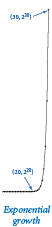One legend says that the world will end when a certain group of monks solves the Towers of Hanoi problem in a temple with 64 golden discs on three diamond needles. We can estimate the amount of time until the end of the world (assuming that the legend is true). If we define the function T(n) to be the number of move directives issued by `towersofhanoi.py` to move n discs from one peg to another, then the recursive code implies that T(n) must satisfy the following equation:

T(n) = 2 T(n - 1) + 1 for n > 1, with T(1) = 1

Such an equation is known in discrete mathematics as a recurrence relation. We can often use them to derive a closed-form expression for the quantity of interest. For example, T(1) = 1, T(2) = 3, T(3) = 7, and T(4) = 15. In general, T(n) = 2n - 1.

Knowing the value of T(n), we can estimate the amount of time required to perform all the moves. If the monks move discs at the rate of one per second, it would take more than one week for them to finish a 20-disc problem, more than 31 years to finish a 30-disc problem, and more than 348 centuries for them to finish a 40-disc problem (assuming that they do not make a mistake). The 64-disc problem would take more than 5.8 billion centuries.

## Gray Code

The playwright Samuel Beckett wrote a play called Quad that had the following property: Starting with an empty stage, characters enter and exit one at a time, but each subset of characters on the stage appears exactly once. The play had four characters and there are 24 = 16 different subsets of four elements; hence the title. How did Beckett generate the stage directions for this play? How would we do it for 5 actors, or more?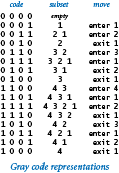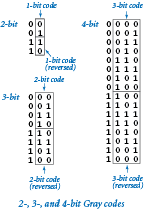An n-bit Gray code is a list of the 2n different n-bit binary numbers such that each entry in the list differs in precisely one bit from its predecessor. Gray codes directly apply to Beckett's problem because we can interpret each bit as denoting whether the integer corresponding to its bit position is in the subset. Changing the value of a bit from 0 to 1 corresponds to an integer entering the subset; changing a bit from 1 to 0 corresponds to and integer exiting the subset.

How do we generate a Gray code? A recursive plan that is very similar to the one that we used for the Towers of Hanoi problem is effective. The n bit binary reflected Gray code is defined recursively as follows:

• the n-1 bit code, with 0 prepended to each word, followed by
• the n-1 bit code in reverse order, with 1 prepended to each word.

The 0-bit code is defined to be null, so the 1-bit code is 0 followed by 1.

The recursive definition leads, after some careful thought, to the implementation in beckett.py for writing Beckett's stage directions.

## Recursive Graphics

Simple recursive drawing schemes can lead to pictures that are remarkably intricate. For example, an H-tree of order n is defined as follows: The base case is null for n = 0. The reduction step is to draw, within the unit square three lines in the shape of the letter H four H-trees of order n-1, one connected to each tip of the H with the additional provisos that the H-trees of order n-1 are centered in the four quadrants of the square, halved in size. Program htree.py takes a command-line argument `n`, and plots an order n H-tree using standard draw.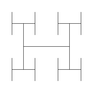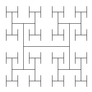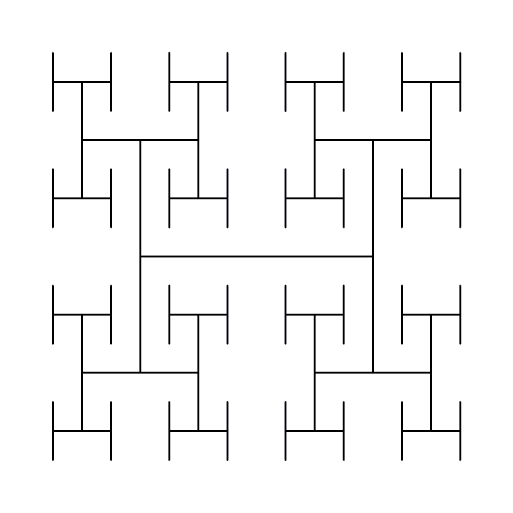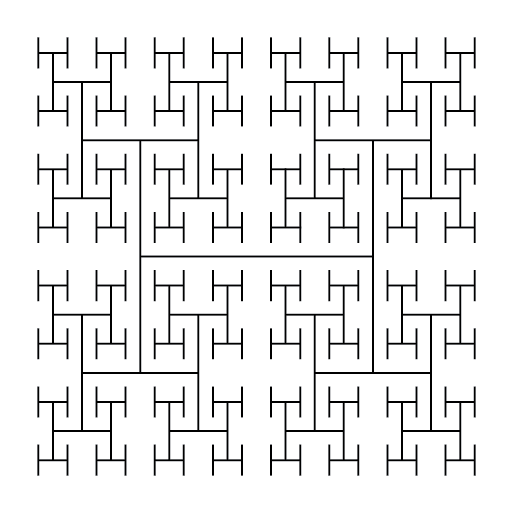## Brownian Bridge

An H-tree is a simple example of a fractal: a geometric shape that can be divided into parts, each of which is (approximately) a reduced size copy of the original. The study of fractals plays an important and lasting role in artistic expression, economic analysis, and scientific discovery. Artists and scientists use them to build compact models of complex shapes that arise in nature and resist description using conventional geometry, such as clouds, plants, mountains, riverbeds, human skin, and many others. Economists also use fractals to model function graphs of economic indicators.

Program brownian.py produces a function graph that approximates a simple example known as a Brownian bridge and closely related functions. You can think of this graph as a random walk that connects two points, from (x0, y0) to (x1, y1), controlled by a few parameters. The implementation is based on the midpoint displacement method, which is a recursive plan for drawing the plot within an interval [x0, x1]. The base case (when the size of the interval is smaller than a given tolerance) is to draw a straight line connecting the two endpoints. The reduction case is to divide the interval into two halves, proceeding as follows:

• Compute the midpoint (xm, ym) of the interval.
• Add to the `y`-coordinate of the midpoint a random value δ, chosen from the Gaussian distribution with mean 0 and given variance.
• Recur on the subintervals, dividing the variance by a given scaling factor s.

The shape of the curve is controlled by two parameters: the volatility (initial value of the variance) controls the distance the graph strays from the straight line connecting the points, and the Hurst exponent controls the smoothness of the curve. We denote the Hurst exponent by H and divide the variance by 22H at each recursive level. When H is 1/2 (divide by 2 at each level) the standard deviation is constant throughout the curve: in this situation, the curve is a Brownian bridge. These images show the output generated by the commands `python brownian.py 1`, `python brownian.py .5`, and `python brownian.py .05`.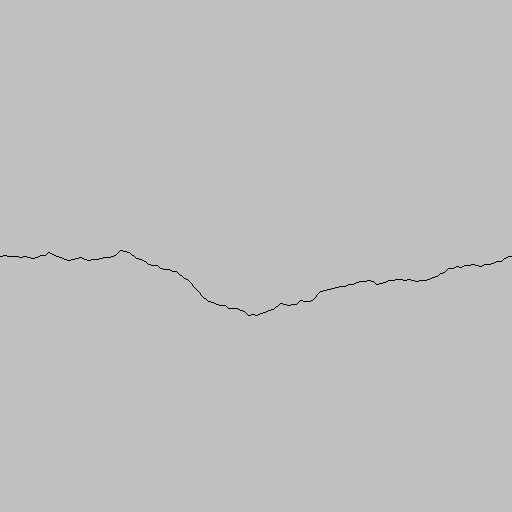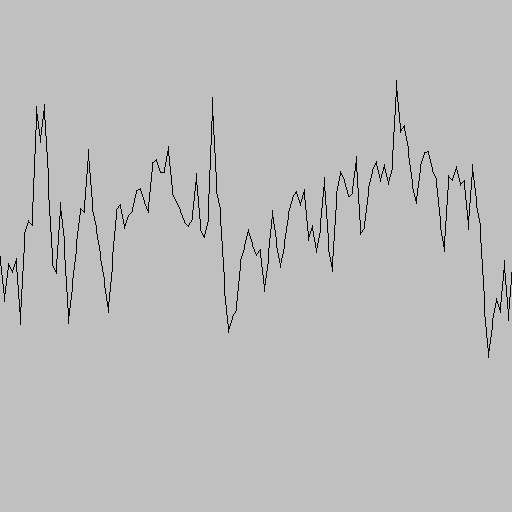## Pitfalls of Recursion

With recursion, you can compose compact and elegant programs that fail spectacularly at runtime.

Missing base case. This recursive function is supposed to compute Harmonic numbers, but is missing a base case:

 ```def H(n): return H(n-1) + 1.0/n; ```

If you call this function, it will repeatedly call itself and never return.

No guarantee of convergence. Another common problem is to include within a recursive function a recursive call to solve a subproblem that is not smaller. For example, this recursive function will go into an infinite recursive loop if it is invoked with an argument n having any value other than 1:

 ```def H(n): if n == 1: return 1.0 return H(n) + 1.0/n ```

Excessive space requirements. Python needs to keep track of each recursive call to implement the function abstraction as expected. If a function calls itself recursively an excessive number of times before returning, the space required by Python for this task may be prohibitive. For example, this recursive function correctly computes the nth harmonic number. However, we cannot use it for large n because the recursive depth is proportional to n, and this creates a `StackOverflowError`.

 ```def H(n): if n == 0: return 0.0 return H(n-1) + 1.0/n ```Excessive recomputation. The temptation to write a simple recursive program to solve a problem must always be tempered by the understanding that a simple program might require exponential time (unnecessarily), due to excessive recomputation. For example, the Fibonacci sequence

 ```0 1 1 2 3 5 8 13 21 34 55 89 144 233 377 ... ```

is defined by the formula Fn = Fn-1 + Fn-2 for n ≥ 2 with F0 = 0 and F1 = 1.

A novice programmer might implement this recursive function to compute numbers in the Fibonacci sequence:

 ```def fib(n): if n == 0: return 0 if n == 1: return 1 return fib(n-1) + fib(n-2) ```

However, this program is spectacularly inefficient! For example, consider what the function does to compute `fib(7)` = 13. It first computes `fib(6)` = 8 and `fib(5)` = 5. To compute `fib(6)`, it recursively computes `fib(5)` = 5 again and `fib(4)` = 3. Things rapidly get worse because both times it computes `fib(5)`, it ignores the fact that it already computed `fib(4)`, and so forth. The number of times this program computes `fib(1)` when computing `fib(n)` is precisely Fn. The mistake of recomputation is compounded, exponentially. No imaginable computer will ever be able to do that many calculations.

Incidentally, a systematic technique known as memoization allows us to avoid this pitfall while still taking advantage of the compact recursive description of a computation. In memoization, we maintain an array that keeps track of the values we have computed so that we can return those values and make recursive calls only for new values. This technique is a form of dynamic programming, a well-studied technique for organizing computations that you will learn if you take courses in algorithms or operations research.

#### Q & A

Q. Are there situations when iteration is the only option available to address a problem?

A. No, any loop can be replaced by a recursive function, though the recursive version might require excessive memory.

Q. Are there situations when recursion is the only option available to address a problem?

A. No, any recursive function can be replaced by an iterative counterpart. In Section 4.3, we will see how compilers produce code for function calls by using a data structure called a stack.

Q. Which should I prefer, recursion or iteration?

A. Whichever leads to the simpler, more easily understood, or more efficient code.

Q. I get the concern about excessive space and excessive recomputation in recursive code. Anything else to be concerned about?

A. Be extremely wary of creating arrays in recursive code. The amount of space used can pile up very quickly, as can the amount of time required for memory management.

#### Exercises

1. What happens if you run `factorial()` with negative value of `n`? With a large value, say 35?

2. Compose a recursive program that computes the value of ln(n!).

3. Give the sequence of integers written by a call to `ex233(6)`:

 ```def ex233(n): if n <= 0: return stdio.writeln(n) ex233(n-2) ex233(n-3) stdio.writeln(n) ```
4. Give the value of `ex234(6)`:

 ```def ex234(n): if n <= 0: return '' return ex234(n-3) + str(n) + ex234(n-2) + str(n) ```
5. Criticize the following recursive function:

 ```def ex235(n): s = ex233(n-3) + str(n) + ex235(n-2) + str(n) if n <= 0: return '' return s ```

Solution: The base case will never be reached. A call to `ex235(3)` will result in calls to `ex235(0)`, `ex235(-3)`, `ex235(-6)`, and so forth until the "maximum depth exceeded" run-time error occurs.

6. Given four positive integers `a`, `b`, `c`, and `d`, explain what value is computed by `gcd(gcd(a, b), gcd(c, d))`.

7. Explain in terms of integers and divisors the effect of the following Euclid-like function.
 ```def gcdlike(p, q): if q == 0: return p == 1 return gcdlike(q, p % q) ```

Solution. Returns whether `p` and `q` are relatively prime.

8. Consider the following recursive function:

 ```def mystery(a, b): if b == 0: return 0 if b % 2 == 0: return mystery(a+a, b//2) return mystery(a+a, b//2) + a ```

What are the values of `mystery(2, 25)` and `mystery(3, 11)`? Given positive integers `a` and `b`, describe what value `mystery(a, b)` computes. Answer the same question, but replace `+` with `*` and replace `return 0` with `return 1`.

Solution. 50 and 33. It computes `a*b`. If you replace `+` with `*`, it computes `ab`.

9. Compose a recursive program `ruler.py` to plot the subdivisions of a ruler using `stddraw` as in the ruler.py program from Section 1.2.

10. Solve the following recurrence relations, all with T(1) = 1. Assume n is a power of two.

• T(n) = T(n/2) + 1
• T(n) = 2T(n/2) + 1
• T(n) = 2T(n/2) + n
• T(n) = 4T(n/2) + 3
11. Prove by induction that the minimum possible number of moves needed to solve the Towers of Hanoi puzzle satisfies the same recurrence as the number of moves used by our recursive solution.

12. Prove by induction that the recursive program given above makes exactly Fn recursive calls to `fib(1)` when computing `fib(n)`.

13. Prove that the second argument to `gcd()` decreases by at least a factor of two for every second recursive call, then prove that `gcd(p, q)` uses at most log2n recursive calls, where n is the larger of p and q.

14. Modify `htree.py` to animate the drawing of the H-tree.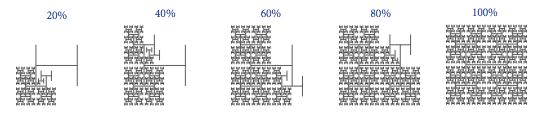Next, rearrange the order of the recursive calls (and the base case), view the resulting animation, and explain each outcome.

#### Creative Exercises

1. Binary representation. Compose a program that takes a positive integer n (in decimal) from the command line and writes its binary representation. Recall that in Section 1.3 we used the method of subtracting out powers of 2. Instead, use the following simpler method: repeatedly divide 2 into n and read the remainders backwards. First, compose a `while` loop to carry out this computation and write the bits in the wrong order. Then, use recursion to write the bits in the correct order.

Solution: See binaryconverter.py.

2. A4 paper. The width-to-height ratio of paper in the ISO format is the square root of 2 to 1. Format A0 has an area of 1 square meter. Format A1 is A0 cut with a vertical line into two equal halves, A2 is A1 cut with a horizontal line into in two halves, and so on. Write a program that takes a command-line argument n and uses `stddraw` to show how to cut a sheet of A0 paper into 2n pieces. Here's a nice illustration of A size formats.

3. Permutations. Compose a program that takes a command-line argument n and writes all n! permutations of the n letters starting at a (assume that `n` is no greater than 26). A permutation of n elements is one of the n! possible orderings of the elements. As an example, when n = 3 you should get the following output. Do not worry about the order in which you enumerate them.

 ```bca cba cab acb bac abc ```

Solution: See permutations.py.

4. Permutations of size k. Modify your solution to the previous exercise so that it takes two command-line arguments n and k, and writes all P(n, k) = n! / (n-k)! permutations that contain exactly k of the n elements. Below is the desired output when k = 2 and n = 4. You need not write them in any particular order.

 ```ab ac ad ba bc bd ca cb cd da db dc ```
5. Solution: See perm.py.

6. Combinations. Compose a program that takes one integer command-line argument n and writes all 2n combinations of any size. A combination is a subset of the n elements, independent of order. As an example, when n = 3 you should get the following output.
 ``` a ab abc ac b bc c ```

Note that the first element written is the empty string (subset of size 0).

Solution: See combinations.py.

7. Combinations of size `k`. Modify your solution to the previous exercise so that it takes two command-line arguments n and k, and writes all C(n, k) = n! / (k! * (n-k)!) combinations of size k. For example, when n = 5 and k = 3 you should get the following output.

 ```abc abd abe acd ace ade bcd bce bde cde ```

Solution: See comb.py.

8. Hamming distance. The Hamming distance between two bit strings of length n is equal to the number of bits in which the two strings differ. Compose a program that takes an integer k and a bit string s from the command line, and writes all bit strings that have Hamming distance at most k from s. For example if k is 2 and s is 0000 then your program should write:

 ```0011 0101 0110 1001 1010 1100 ```

Hint: choose k of the n bits in s to flip.

9. Recursive squares. Compose a program to produce each of the following recursive patterns. The ratio of the sizes of the squares is 2.2:1. To draw a shaded square, draw a filled gray square, then an unfilled black square.

1.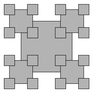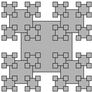2.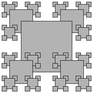3.4.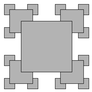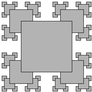Solution: See recursivesquares.py for a solution to part a.

10. Pancake flipping. You have a stack of n pancakes of varying sizes on a griddle. Your goal is to rearrange the stack in descending order so that the largest pancake is on the bottom and the smallest one is on top. You are only permitted to flip the top k pancakes, thereby reversing their order. Devise a scheme to arrange the pancakes in the proper order by using at most 2n - 3 flips.

Hint: you can try out strategies here.

11. Gray code. Modify `beckett.py` to write the Gray code, not just the sequence of bit positions that change.

Solution: See graycode.py.

12. Towers of Hanoi variant. Consider the following variant of the Towers of Hanoi problem. There are 2n discs of increasing size stored on three poles. Initially all of the discs with odd size (1, 3, ..., 2n-1) are piled on the left pole from top to bottom in increasing order of size; all of the discs with even size (2, 4, ..., 2n) are piled on the right pole. Compose a program to provide instructions for moving the odd discs to the right pole and the even discs to the left pole, obeying the same rules as for Towers of Hanoi.

13. Animated Towers of Hanoi. Compose a program that uses `stddraw` to animate a solution to the Towers of Hanoi problem, moving the discs at a rate of approximately 1 per second.

Solution: See animatedhanoi.py.

14. Sierpinski triangles. Compose a recursive program to draw the Sierpinski gasket. As with `htree.py`, use a command-line argument to control the depth of recursion.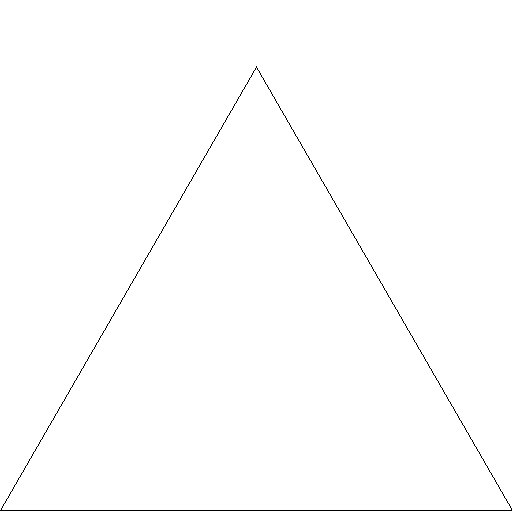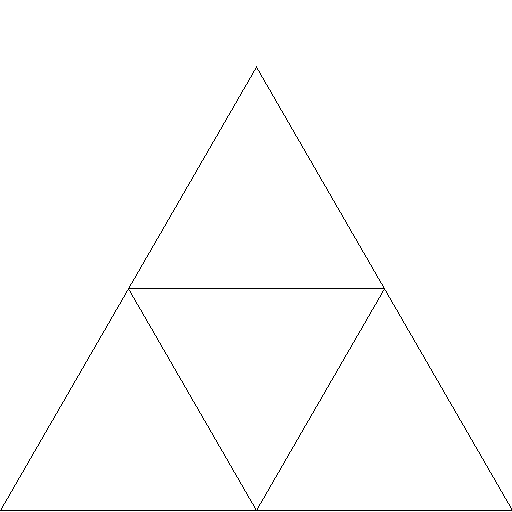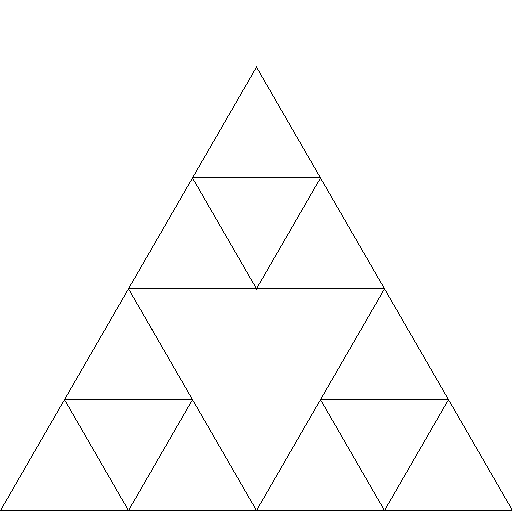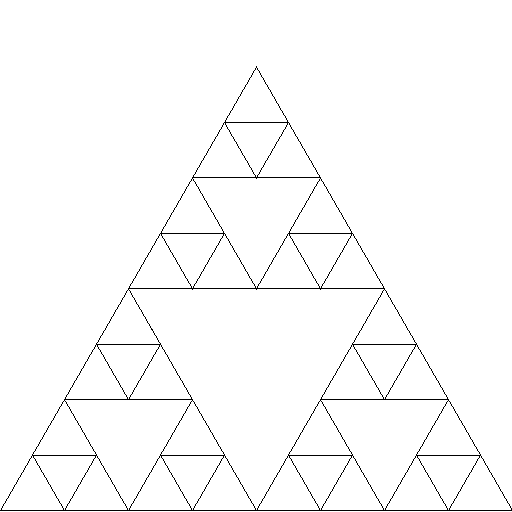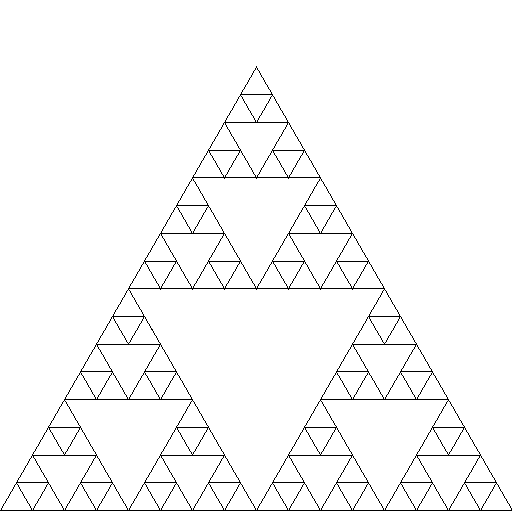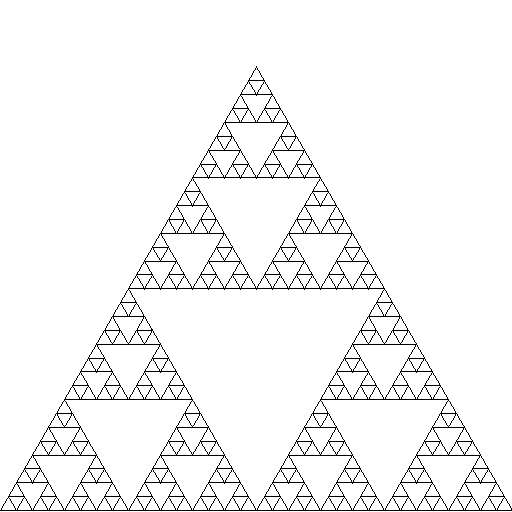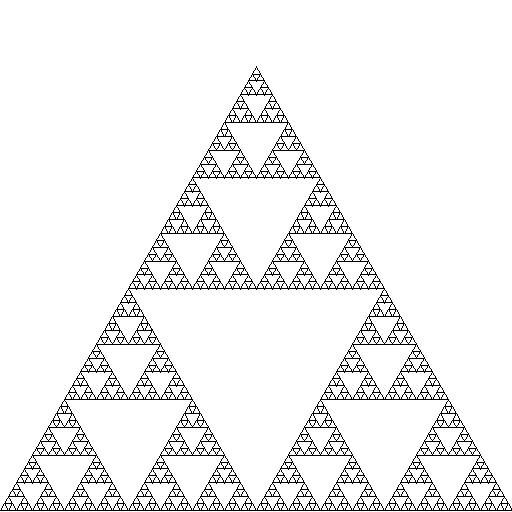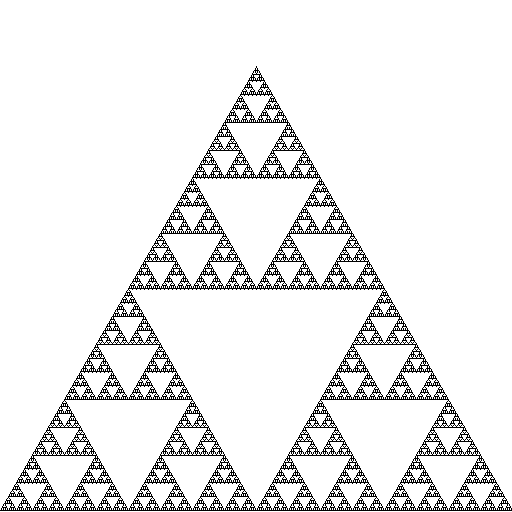15. Binomial distribution. Estimate the number of recursive calls that would be used by the code

 ```def binomial(n, k): if (n == 0) or (k < 0): return 1.0 return (binomial(n-1, k) + binomial(n-1, k-1)) / 2.0 ```

to compute `binomial(100, 50)`. Develop a better implementation that is based on memoization. Hint: see the Binomial coefficients exercise in Section 1.4.

16. A strange function. Consider McCarthy's 91 function:

 ```def mcCarthy(n): if n > 100: return n - 10 return mcCarthy(mcCarthy(n+11)) ```

Determine the value of `mcCarthy(50)` without using a computer. Give the number of recursive calls used by `mcCarthy()` to compute this result. Either prove that the base case is reached for all positive integers n or give a value of n for which this function goes into an infinite recursive loop.

17. Collatz function. Consider the following recursive function in collatz.py, which is related to a famous unsolved problem in number theory, known as the Collatz problem or the 3n + 1 problem.

 ```def collatz(n): stdio.write(str(n) + ' ') if n == 1: return elif n % 2 == 0: collatz(n // 2) else: collatz(3*n + 1) ```

For example, a call to `collatz(7)` writes the sequence of 17 integers

 ```7 22 11 34 17 52 26 13 40 20 10 5 16 8 4 2 1 ```

after 17 function calls. Compose a program that takes a command-line argument m and returns the value of n < m for which the number of recursive calls for `collatz(n)` is maximized. The unsolved problem is that no one knows whether the function terminates for all positive values of n (mathematical induction is no help because one of the recursive calls is for a larger value of the argument). Develop a better implementation that is based on memoization.

18. Recursive tree. Compose a program that takes a command-line argument n and produces tree-like recursive patterns like these for n equal to 1, 2, 3, 4, and 8: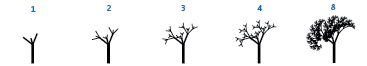19. Brownian island. Benoit Mandelbrot asked the famous question How long is the coast of Britain? Modify `brownian.py` to compose a program that plots Brownian islands, whose coastlines resemble that of Great Britain. The modifications are simple: first, change `curve()` to add a gaussian to the x coordinate as well as to the y coordinate; second, change `main()` to draw a curve from the point at the center of the canvas back to itself. Experiment with various values of the arguments to get your program to produce islands with a realistic look.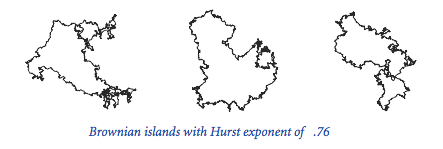Solution: See brownianisland.py.